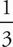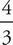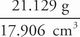# SAT Math Multiple Choice Question 60: Answer and Explanation

### Test Information

Question: 60

6. A toy pyramid (not shown) is made from poly(methyl methacrylate), better known by its trade term Lucite. The toy pyramid has a regular hexagonal base of 15 cm2 and a height of 4 cm. In the base of the pyramid, there is a semispherical indentation 2 cm in diameter. If the pyramid weighs 21.129 g, then what is the density of Lucite? (Density equals mass divided by volume.)

• A. 1.06 g/cm3
• B. 1.18 g/cm3
• C. 2.09 g/cm3
• D. 6.51 g/cm3

B Work the problem in steps. You are given the mass, so to find density you need to find the volume of the pyramid. The formula at the beginning of the section tells you that, for a pyramid, V =Bh, where B is the area of the base of the pyramid and h is the height. Therefore, the volume of the pyramid is(15)(4) = 20. However, you need to subtract the volume of the semispherical indentation in the base. Once again, the reference sheet found beginning of the Math section tells you thatthe volume of a sphere is given by the equation V =πr3. Because the diameter of the indentation is 2 cm, the radius of the hemisphere is 1 cm. If it were a whole sphere, the volume of the indentation would beπ(1)3 = 4.189; you want only half, so dividing by 2 gives you 2.094 cm3 for the hemisphere. Subtracting 2.094 cm3 from the 20 cm3 of the pyramid gives you a total volume of 20 - 2.094 = 17.906 cm3. Finally, you can find the density of Lucite by using the definition of density: Density =≈ 1.18 g/cm3, which is (B).